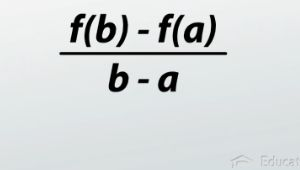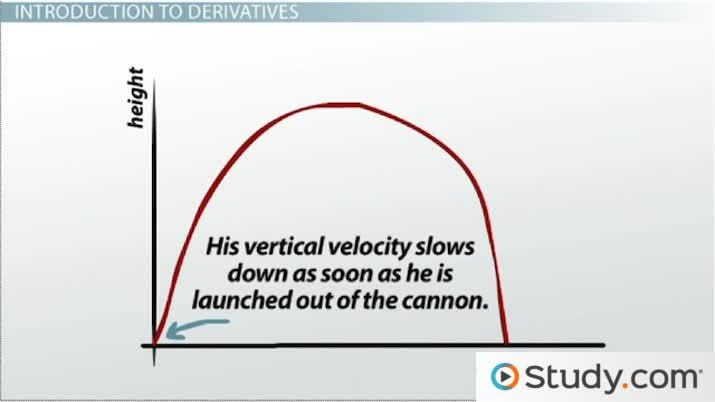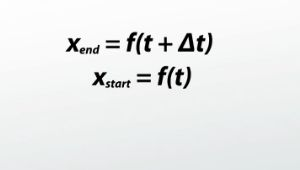# Derivatives: The Formal Definition

Lesson Transcript
Instructor: Jeff Calareso

Jeff teaches high school English, math and other subjects. He has a master's degree in writing and literature.

In calculus, the derivative is defined as a function's instantaneous rate of change. Deepen your understanding of derivatives and learn how to write equations to find the derivative at begin and end times. Updated: 08/09/2021

## Introduction to DerivativesLet's take a look at Super C. Super C's height as a function of time looks a little bit like this. We know that Super C's velocity slows down as soon as he is launched from the cannon - at least his velocity in the vertical direction. His velocity at any given point in time is his instantaneous rate of change. Well, in calculus, we call that the derivative. The derivative is a function for the instantaneous rate of change. So how do we find this derivative? Well, what is the instantaneous rate of change? We know that the average velocity, the average rate of change, is the end point minus the start point, divided by the region: (f(b) - f(a))/(b - a). So this is the change in location divided by the change in time.

Well, that's the average over some long time period. But maybe we should look at it over a very short time period. Maybe, to make this an instantaneous rate of change, that time period is really zero. To make this an instantaneous rate of change, we're going to look at the limit as the change in time goes to zero - so, as our little region of time that we care about goes to zero.An error occurred trying to load this video.

Try refreshing the page, or contact customer support.

Coming up next: Derivatives: Graphical Representations

### You're on a roll. Keep up the good work!

Replay
Your next lesson will play in 10 seconds
• 0:06 Introduction to Derivatives
• 1:32 Writing Derivatives
• 3:39 Lesson Summary
Save Save

Want to watch this again later?

Timeline
Autoplay
Autoplay
Speed SpeedTo unlock this lesson you must be a Study.com Member.

### Register to view this lesson

Are you a student or a teacher?